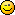<![CDATA[Math Is Fun Forum / divide]]> 2006-11-10T18:51:28Z FluxBB https://www.mathisfunforum.com/viewtopic.php?id=4986 <![CDATA[Re: divide]]> You're doing it as if the dividend has an x² in it, when it actually has an x³.

x² -3x +11 r -34.
-------------------------
x+3 | x³ +2x -1
x³ +3x²
--------------------
-3x² +2x -1
-3x² -9x
--------------------
11x -1
11x +33
----------
-34

So I get that (x³ +2x -1)/(x+3) = x² -3x +11, with a remainder of -34.

A quick check is to put x=-3 into the dividend and see if we get the remainder.

(-3)³ +2(-3) -1 = -27-6-1 = -34, so the answer is probably right.

]]>
https://www.mathisfunforum.com/profile.php?id=641 2006-11-10T18:51:28Z https://www.mathisfunforum.com/viewtopic.php?pid=48922#p48922
<![CDATA[Re: divide]]> ok

x+3 / x^3 + 2x - 1

x - 1
-------------------
x + 3  | x^3 + 2x - 1
x^3 + 3x
----------------
5x - 1
-1x - 3
----------------
+1x + 3
--------------
6x + (-2)
now what do i do? and please explain it well to me how to do it if i did this wrong!

]]>
https://www.mathisfunforum.com/profile.php?id=5209 2006-11-10T15:25:33Z https://www.mathisfunforum.com/viewtopic.php?pid=48889#p48889
<![CDATA[Re: divide]]> Yes, x^3 = x cubed.]]>
https://www.mathisfunforum.com/profile.php?id=4454 2006-11-10T07:17:23Z https://www.mathisfunforum.com/viewtopic.php?pid=48872#p48872
<![CDATA[Re: divide]]> x^3 is it read as x raised to 3??

you are dividing polynomials.. right?
i think that is not the right way to divide it..

oh.. i have to get my pencil..

]]>
https://www.mathisfunforum.com/profile.php?id=5611 2006-11-10T05:51:46Z https://www.mathisfunforum.com/viewtopic.php?pid=48862#p48862
<![CDATA[divide]]> divide
x^3 + 2x - 1 by x + 3

so

x^3 + 2x - 1
---------------
x + 3

x^3 + 3x - 2

the way i did it ..it seems to me that i did it wrong
can you correct me on this?

]]>
https://www.mathisfunforum.com/profile.php?id=5209 2006-11-09T14:37:30Z https://www.mathisfunforum.com/viewtopic.php?pid=48767#p48767